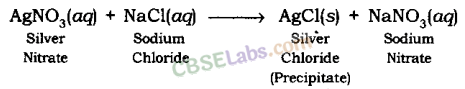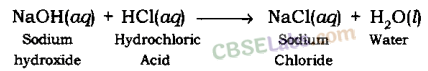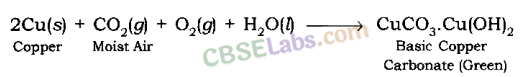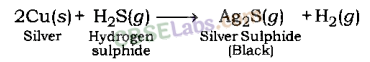CBSE Class 10 Science Notes Chapter 1 Chemical Reactions and Equations Pdf free download is part of Class 10 Science Notes for Quick Revision. Here we have given NCERT Class 10 Science Notes Chapter 1 Chemical Reactions and Equations. https://meritbatch.com/chemical-reactions-and-equations-class-10-notes/

According to new CBSE Exam Pattern, MCQ Questions for Class 10 Science pdf Carries 20 Marks.

## CBSE Class 10 Science Notes Chapter 1 Chemical Reactions and Equations

Chemical Reactions And Equations Class 10 Notes
Chemical Reactions and Equations:
Balanced and unbalanced chemical equations and balancing of chemical equations.

### What is a chemical reaction Class 10?

Chemical Reaction: The transformation of chemical substance into another chemical substance is known as Chemical Reaction. For example: Rusting of iron, the setting of milk into curd, digestion of food, respiration, etc.

In a chemical reaction, a new substance is formed which is completely different in properties from the original substance, so in a chemical reaction, a chemical change takes place.
Only a rearrangement of atoms takes place in a chemical reaction.

• The substances which take part in a chemical reaction are called reactants.
• The new substances produced as a result of a chemical reaction are called products.

Example: The burning of magnesium in the air to form magnesium oxide is an example of a chemical reaction.
2Mg(s) + O2(g)$\underrightarrow { \triangle }$ 2MgO(s)
Before burning in air, the magnesium ribbon is cleaned by rubbing with sandpaper.
This is done to remove the protective layer of basic magnesium carbonate from the surface of the magnesium ribbon.

Chemical Reaction And Equation Class 10 Notes
Reactant:
Substances which take part in a chemical reaction are called reactants.
Example: Mg and O2.

Product: New substance formed after a chemical reaction is called a product.
Example: MgO.

Class 10 Science Chapter 1 Notes
Characteristics of Chemical Reactions :

(i) Evolution of gas: The chemical reaction between zinc and dilute sulphuric acid is characterised by the evolution of hydrogen gas.
Zn(s) + H2SO4(aq) → ZnSO4(aq) + H2(g) ↑

(ii) Change in Colour: The chemical reaction between citric acid and purple coloured potassium permanganate solution is characterised by a change in colour from purple to colourless.
The chemical reaction between sulphur dioxide gas and acidified potassium dichromate solution is characterized by a change in colour from orange to green.

(iii) Change in state of substance: The combustion reaction of candle wax is characterised by a change in state from solid to liquid and gas (because the wax is a solid, water formed by the combustion of wax is a liquid at room temperature whereas, carbon dioxide produced by the combustion of wax is a gas). There are some chemical reactions which can show more than one characteristics.

(iv) Change in temperature: The chemical reaction between quick lime water to form slaked lime is characterized by a change in temperature (which is a rise in temperature).
The chemical reaction between zinc granules and dilute sulphuric acid is also characterised by a change in temperature (which is a rise in temperature).

Class 10 Chemistry Chapter 1 Notes
(v) Formation of precipitate:
The chemical reaction between sulphuric acid and barium chloride solution is characterised by the formation of a white precipitate of barium sulphate.
BaCl2(aq) + H2SO4(aq) → BaSO4(s) (ppt) + 2HCl(aq)

### What is a chemical Equation Class 10?

Chemical Equation: Representation of chemical reaction using symbols and formulae of the substances is called Chemical Equation.
Example: A + B → C + D
In this equation, A and B are called reactants and C and D are called the products. The arrow shows the direction of the chemical reaction. Condition, if any, is written generally above the arrow.

When hydrogen reacts with oxygen, it gives water. This reaction can be represented by the following chemical equation:
Hydrogen + Oxygen → Water
H2 + O2 → H2O
In the first equation, words are used and in second, symbols of substances are used to write the chemical equation. For convenience, the symbol of substance is used to represent chemical equations.
A chemical equation is a way to represent the chemical reaction in a concise and informative way.
A chemical equation can be divided into two types: Balanced Chemical Equation and Unbalanced Chemical Equation.

Chemical Reaction And Equation
(a) Balanced Chemical Equation:
A balanced chemical equation has the number of atoms of each element equal on both sides.
Example: Zn + H2SO4 → ZnSO4 + H2
In this equation, numbers of zinc, hydrogen and sulphate are equal on both sides, so it is a Balanced Chemical Equation.
According to the Law of Conservation of Mass, mass can neither be created nor destroyed in a chemical reaction. To obey this law, the total mass of elements present in reactants must be equal to the total mass of elements present in products.

Chemical Reactions And Equations
(b) Unbalanced Chemical Equation:
If the number of atoms of each element in reactants is not equal to the number of atoms of each element present in the product, then the chemical equation is called Unbalanced Chemical Equation.
Example: Fe + H2O → Fe3O4 + H2
In this example, a number of atoms of elements are not equal on two sides of the reaction. For example; on the left-hand side only one iron atom is present, while three iron atoms are present on the right-hand side. Therefore, it is an unbalanced chemical equation.

Balancing a Chemical Equation: To balance the given or any chemical equation, follow these steps:
Fe + H2O → Fe3O4 + H2
Write the number of atoms of elements present in reactants and in products in a table as shown here.

 Name of atom No. of atoms in the reactant No. of atoms in the product Iron 1 3 Hydrogen 2 2 Oxygen 1 4

Balance the atom which is maximum in number on either side of a chemical equation.
In this equation, the number of oxygen atom is the maximum on the RHS.
To balance the oxygen, one needs to multiply the oxygen on the LHS by 4, so that, the number of oxygen atoms becomes equal on both sides.
Fe + 4 × H2O → Fe3O4 + H2
Now, the number of hydrogen atoms becomes 8 on the LHS, which is more than that on the RHS. To balance it, one needs to multiply the hydrogen on the RHS by 4.
Fe + 4 × H2O → Fe3O4 + 4 × H2
After that, the number of oxygen and hydrogen atoms becomes equal on both sides. The number of iron is one on the LHS, while it is three on the RHS. To balance it, multiply the iron on the LHS by 3.
3 × Fe + 4 × H2O → Fe3O4 + 4 × H2
Now the number of atoms of each element becomes equal on both sides. Thus, this equation becomes a balanced equation.

 Name of atom No. of atoms in the reactant No. of atoms in the product Iron 3 3 Hydrogen 8 8 Oxygen 4 4

After balancing, the above equation can be written as follows:
3Fe + 4H2O → Fe3O4 + 4H2.

Chemical Reactions And Equations Class 10 Notes Pdf

Writing the symbols of physical states of substances in a chemical equation:
By writing the physical states of substances, a chemical equation becomes more informative.

• Gaseous state is represented by symbol (g).
• Liquid state is represented by symbol (l).
• Solid state is written by symbol (s).
• Aqueous solution is written by symbol (aq).
• Writing the condition in which reaction takes place: The condition is generally written above and/or below the arrow of a chemical equation.

Thus, by writing the symbols of the physical state of substances and condition under which reaction takes place, a chemical equation can be made more informative.

### What are the types of a chemical reaction Class 10?

Ch 1 Science Class 10 Notes
Types of Chemical Reactions:
Combination Reaction, Decomposition Reaction, Displacement Reaction, Double Displacement Reaction, Neutralization Reactions, Exothermic – Endothermic Reactions and Oxidation-Reduction Reactions.

Types of Chemical Reactions:
Chemical reactions can be classified in following types:
(i) Combination Reaction: Reactions in which two or more reactants combine to form one product are called Combination Reactions.
A general combination reaction can be represented by the chemical equation given here:
A + B → AB
Examples:
When magnesium is burnt in the air (oxygen), magnesium oxide is formed. In this reaction, magnesium is combined with oxygen.
Mg(s) + O2(g) → 2MgO(s)
Magnesium + Oxygen → Magnesium Oxide

When carbon is burnt in oxygen (air), carbon dioxide is formed. In this reaction, carbon is combined with oxygen.
C (s) + O2(g) → CO2(g)
Carbon + Oxygen → Carbon dioxide

(ii) Decomposition Reaction: Reactions in which one compound decomposes in two or more compounds or elements are known as Decomposition Reaction. A decomposition reaction is just the opposite of combination reaction.
A general decomposition reaction can be represented as follows :
AB → A + B
Examples:
When calcium carbonate is heated, it decomposes into calcium oxide and carbon dioxide.
CaCO3(s)$\underrightarrow { heat }$ CaO(s) + CO2(g)
Calcium carbonate → Calcium oxide + Carbon dioxide

When ferric hydroxide is heated, it decomposes into ferric oxide and water
2Fe(OH)3(s)$\underrightarrow { \triangle }$ Fe2O3(s) + 3H2O(l)

Chemistry Class 10 Chapter 1 Notes
Thermal Decomposition:
The decomposition of a substance on heating is known as Thermal Decomposition.
Example: 2Pb(NO3)2(s)$\underrightarrow { heat }$ 2PbO(s) + 4NO2(g) + O2(g)

Electrolytic Decomposition: Reactions in which compounds decompose into simpler compounds because of passing of electricity, are known as Electrolytic Decomposition. This is also known as Electrolysis.
Example: When electricity is passed in water, it decomposes into hydrogen and oxygen.
2H2O(l)$\xrightarrow [ Electrolysis ]{ Electric\quad Current }$ 2H2(g) + O2(g)

Photolysis or Photo Decomposition Reaction: Reactions in which a compound decomposes because of sunlight are known as Photolysis or Photo Decomposition Reaction.
Example: When silver chloride is put in sunlight, it decomposes into silver metal and chlorine gas.
2AgCl(s) (white)$\underrightarrow { Sunlight }$ 2Ag(s) (grey) + Cl2(g)

Photographic paper has a coat of silver chloride, which turns into grey when exposed to sunlight. It happens because silver chloride is colourless while silver is a grey metal.

Class 10 Science Notes Chapter 1
(iii) Displacement Reaction:
The chemical reactions in which a more reactive element displaces a less reactive element from a compound is known as Displacement Reactions. Displacement reactions are also known as Substitution Reaction or Single Displacement/ replacement reactions.
A general displacement reaction can be represented by using a chemical equation as follows :
A + BC → AC + B
Displacement reaction takes place only when ‘A’ is more reactive than B. If ‘B’ is more reactive than ‘A’, then ‘A’ will not displace ‘C’ from ‘BC’ and reaction will not be taking place.
Examples:
When zinc reacts with hydrochloric acid, it gives hydrogen gas and zinc chloride.
Zn(s) + 2HCl(aq) → ZnCl2(aq) + H2(g)

When zinc reacts with copper sulphate, it forms zinc sulphate and copper metal.
Zn(s) + CuSO4(aq) → ZnSO4(aq) + Cu(s)

Chemical Reaction And Equation Class 10
(iv) Double Displacement Reaction:
Reactions in which ions are exchanged between two reactants forming new compounds are called Double Displacement Reactions.
AB + CD → AC + BD
Examples:
When the solution of barium chloride reacts with the solution of sodium sulphate, white precipitate of barium sulphate is formed along with sodium chloride.
BaCl2(aq) + Na2SO4(aq) → BaSO4(s) (Precipitate) + 2NaCl(aq)

When sodium hydroxide (a base) reacts with hydrochloric acid, sodium chloride and water are formed.
NaOH(aq) + HCl(aq) → NaCl(aq) + H2O(l)

Note: Double Displacement Reaction, in which precipitate is formed, is also known as precipitation reaction. Neutralisation reactions are also examples of double displacement reaction.

Science Class 10 Chapter 1 Notes
Precipitation Reaction:
The reaction in which precipitate is formed by the mixing of the aqueous solution of two salts is called Precipitation Reaction.
Example:Neutralization Reaction: The reaction in which an acid reacts with a base to form salt and water by an exchange of ions is called Neutralization Reaction.
Example:(v) Oxidation and Reduction Reactions:
Oxidation: Addition of oxygen or non-metallic element or removal of hydrogen or metallic element from a compound is known as Oxidation.
Elements or compounds in which oxygen or non-metallic element is added or hydrogen or metallic element is removed are called to be Oxidized.
Reduction: Addition of hydrogen or metallic element or removal of oxygen or non-metallic element from a compound is called Reduction.
The compound or element which goes under reduction in called to be Reduced.
Oxidation and Reduction take place together.
Oxidizing agent:

• The substance which gives oxygen for oxidation is called an Oxidizing agent.
• The substance which removes hydrogen is also called an Oxidizing agent.

Chemical Reaction And Equation Notes
Reducing agent:

• The substance which gives hydrogen for reduction is called a Reducing agent.
• The substance which removes oxygen is also called a Reducing agent.

The reaction in which oxidation and reduction both take place simultaneously is called Redox reaction.
When copper oxide is heated with hydrogen, then copper metal and hydrogen are formed.
CuO + H2 → Cu + H2O
(i) In this reaction, CuO is changing into Cu. Oxygen is being removed from copper oxide. Removal of oxygen from a substance is called Reduction, so copper oxide is being reduced to copper.

(ii) In this reaction, H2 is changing to H2O. Oxygen is being added to hydrogen. Addition of oxygen to a substance is called Oxidation, so hydrogen is being oxidised to water.

• The substance which gets oxidised is the reducing agent.
• The substance which gets reduced is the oxidizing agent.

(vi) Exothermic and Endothermic Reactions:
Exothermic Reaction: Reaction which produces energy is called Exothermic Reaction. Most of the decomposition reactions are exothermic.
Example:
Respiration is a decomposition reaction in which energy is released.When quick lime (CaO) is added to water, it releases energy.Endothermic Reaction: A chemical reaction in which heat energy is absorbed is called Endothermic Reaction.
Example: Decomposition of calcium carbonate.Effects of Oxidation Reactions in Everyday life: Corrosion and Rancidity.
Corrosion: The process of slow conversion of metals into their undesirable compounds due to their reaction with oxygen, water, acids, gases etc. present in the atmosphere is called Corrosion.
Example: Rusting of iron.

Rusting: Iron when reacts with oxygen and moisture forms red substance which is called Rust.The rusting of iron is a redox reaction.
Corrosion (rusting) weakens the iron and steel objects and structures such as railings, car bodies, bridges and ships etc. and cuts short their life.
Methods to Prevent Rusting

• By painting.
• By greasing and oiling.
• By galvanisation.

Class 10 Chemistry Chapter 1 Notes Pdf
Corrosion of Copper:
Copper objects lose their lustre and shine after some time because the surface of these objects acquires a green coating of basic copper carbonate, CuCO3.Cu(OH)2 when exposed to air.Corrosion of Silver Metal: The surface of silver metal gets tarnished (becomes dull) on exposure to air, due to the formation of a coating of black silver sulphide(Ag2S) on its surface by the action of H2S gas present in the air.Rancidity: The taste and odour of food materials containing fat and oil changes when they are left exposed to air for a long time. This is called Rancidity. It is caused due to the oxidation of fat and oil present in food materials.

Methods to prevent rancidity:

• Vacuum packing.
• Replacing air by nitrogen.
• Refrigeration of foodstuff.

Class 10th Chemistry Chapter 1 Notes
1. Chemical Reaction: During chemical reactions, the chemical composition of substances changes or new substances are formed.

2. Chemical Equation: Chemical reactions can be written in chemical equation form which should always be balanced.

3. Types of Chemical Reactions:

Combination reaction: A single product is formed from two or more reactants.
2Mg + O2 → 2MgO

Decomposition reaction: A single reactant breaks down to yield two or more products.

• Thermal decomposition: 2Pb(NO2)2 → 2PbO + 4NO2 + O2
• Electrolysis: 2H20 → 2H2 + O2
• Photochemical reaction: 2AgBr → 2Ag + Br2

Science Chapter 1 Class 10 Notes
Displacement reaction:
One element is displaced by another element.
Zn + CuSO4 → ZnSO4 + Cu

Double displacement reaction: Exchange of ions between reactants.
AgNO3 + NaCl → AgCl + NaNO3

Redox reaction: Both oxidation and reduction take place simultaneously.
CuO + H2 → Cu + H2O

Exothermic reaction: A chemical reaction in which heat energy is evolved.
C + O2 → CO2 (g) + heat

Endothermic reaction: A chemical reaction in which heat energy is absorbed.
ZnCO3 + Heat → ZnO + CO2

Redox reaction: Chemical reaction in which both oxidation and reduction take place simultaneously.

4. Oxidation: Reaction that involves the gain of oxygen or loss of hydrogen.

5. Reduction: Reaction that shows the loss of oxygen or gain of hydrogen.
ZnO + C → Zn + CO
ZnO is reduced to Zn—reduction. C is oxidized to CO—Oxidation.

Chemistry Chapter 1 Class 10 Notes
6. Effects of Oxidation Reactions in Our Daily Life:

• Corrosion: It is an undesirable change that occurs in metals when they are attacked by moisture, air, acids and bases.
Example, Corrosion (rusting) of Iron: Fe2O3. nH2O (Hydrated iron oxide)
• Rancidity: Undesirable change that takes place in oil containing food items due to the oxidation of fatty acids.
Preventive methods of rancidity: Adding antioxidants to the food materials, storing food in the airtight container, flushing out air with nitrogen gas and refrigeration.

We hope the given CBSE Class 10 Science Notes Chapter 1 Chemical Reactions and Equations Pdf free download will help you. If you have any query regarding NCERT Class 10 Science Notes Chapter 1 Chemical Reactions and Equations, drop a comment below and we will get back to you at the earliest.